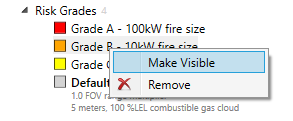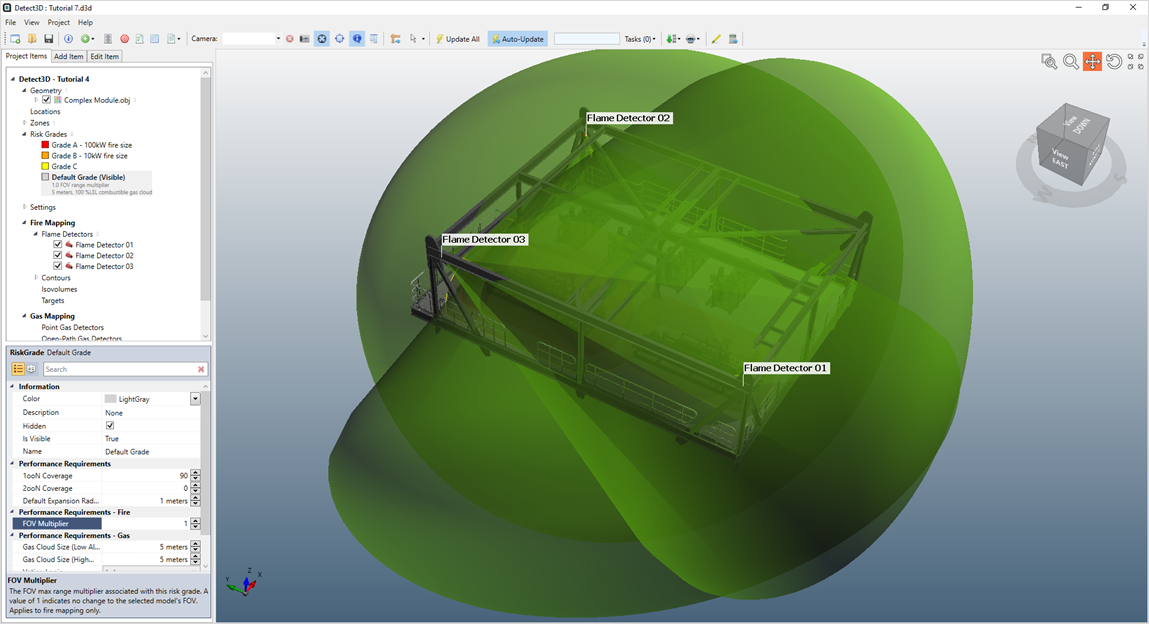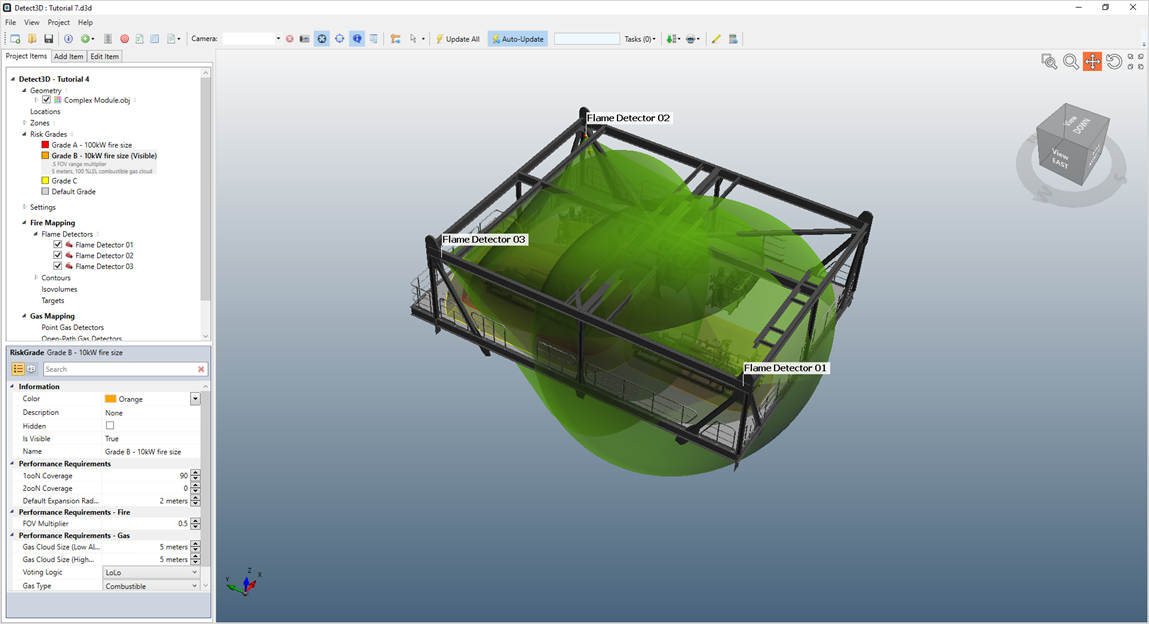# Reviewing Updated Coverage Results

Open the Coverage Results Table and set the Show results by to Subzone. Here there is a difference in the coverage from what was calculated in Tutorial 4. A comparison is shown below. Sub-Zone 01 has a lower coverage results as the FOV Multiplier for the associated risk grade is 0.5 (described below). Sub-Zone 02 with the Grade A - 100kW fire size has little difference as larger FOVs applied to detectors in the same position retain the same blockages throughout the FOV, if the module was bigger a coverage difference may be seen for this sub-zone.

The coverage results for Tutorial 7 will vary by sub-zone this is because there is a different risk grade applied to each. The risk grades with the update FOV Multiplier factors is then applied to the coverage calculation for the associated zone or sub-zones.Tutorial 7 - Figure 8 - Comparison of coverage results before (left) and after (right) FOV Multipliers have been applied

As Sub-Zone 01 has the Grade B - 10kW fire size risk grade applied the resulting coverage will be less as the flame detector FOVs have been reduced by half (the FOV Multiplier of 0.5) to simulate a 10kW fire. This would then lower the calculated coverage as the detector positions have not moved and have a shorter maximum range.

Right-click the Grade B - 10kW fire size in the Project Items tab and select Make Visible as shown in Figure 9 and toggle on the Unobstructed FOVs for the flame detectors.Tutorial 7 - Figure 9 - Changing the Visible Risk Grade in the projectTutorial 7 - Figure 10 - Unobstructed FOVs with the Default Grade Visible - FOV Multiplier = 1Tutorial 7 - Figure 11 - Unobstructed FOVs with the Grade B - 10kW Visible - FOV Multiplier = 0.5

In Figure 10 and 11 we see the smaller FOVs when the Grade B - 10kW fire size risk grade is visible, which explains the coverage calculation for Sub-Zone 01 as some of the flame detectors no longer reach the defined region. You may repeat the process above for the Grade A - 100kW fire size risk grade.

It is important to realize that the 3D window may not appear to have the correct FOV distance. This will always depend on which risk grade is visible and thus which FOV Multiplier is applied to the Visible risk grade. Contours will always display the properties of the Visible risk grade which may be different than was appears in the coverage results table which is dependent on the risk grade of the zone and sub-zone. Meaning that the Visible risk grade could be showing a 0.5 FOV Multiplier in the 3D window but coverage results for a sub-zone in the project will be calculated for a FOV Multiplier of 1.58

This concludes Tutorial 7. You should now have an understanding of FOV multipliers and Detect3D's Inverse Square Law Tool.

When you have finished viewing the results close the project.